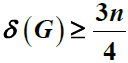# 图中具有指定性质的不交子图Disjoint Subgraphs with Specified Properties in Graphs

DOI: 10.12677/AAM.2017.62016, PDF, HTML, XML, 下载: 892  浏览: 4,719  国家自然科学基金支持

Abstract: Let G be a graph of order n with n≥4k, where k is a positive integer. Suppose that, then the partition of G can be k-1 vertex disjoint 4-cliques and a chordal cycle, where the degree of vertexes in this chordal cycle is equal or greater than 3 or 4.

  Erdös, P. (1967) Extremal Problems in Graph Theory. In: Harary, F., Ed., A Seminar in Graph Theory, Holt, Rinehart and Winston, 54-56.  Bollobás, B. (2004) Extremal Graph Theory. Courier Corporation.  Corradi, K. and Hajnal, A. (1963) On the Maximal Number of Independent Circuits in a Graph. Acta Mathematica Hungarica, 14, 423-439. https://doi.org/10.1007/BF01895727  Wang, H. Covering a Graph with Cycles of Lengths at Least 4.  Ore, O. (1960) Note on Hamilton Circuits. The American Mathematical Monthly, 67, 55. https://doi.org/10.2307/2308928  Chartrand, G., Lesniak, L. and Zhang, P. (2010) Graphs & Digraphs. CRC Press.